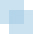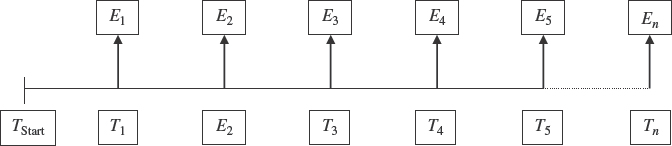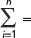# APPENDIX B

##INTRODUCTION

In this appendix, models are developed that calculate the present value of various cash flow streams frequently encountered when valuing business plans. The theoretical framework for these models was developed in Appendix A. Where possible the models refer to the equation(s) in Appendix A that form the basis for the various business models that follow.

##STREAM OF DISTINCT CASH FLOWS1FIGURE B-1 Stream of Distinct Cash Flows

Application

Equation [B-1] can be used to calculate the present value of any stream of cash flows that are based on estimates or growth assumptions for each period. In this sense [B-1] is completely general and in fact is the starting point for developing special cases such as the perpetual and finite models. Equation [B-2] is simply the expanded form of Equation [B-1].

Appendix A Reference:

Equations [A-1] and [A-2]Where:

 PV = Present value of a stream of cash flowsSum of the periodic cash flows in increments ...

Get Corporate Value Creation: An Operations Framework for Nonfinancial Managers now with the O’Reilly learning platform.

O’Reilly members experience books, live events, courses curated by job role, and more from O’Reilly and nearly 200 top publishers.